# Math in Focus Grade 4 Cumulative Review Chapters 3 and 4 Answer Key

Go through the Math in Focus Grade 4 Workbook Answer Key Cumulative Review Chapters 3 and 4 to finish your assignments.

## Math in Focus Grade 4 Cumulative Review Chapters 3 and 4 Answer Key

Concepts and Skills

Multiply. (Lessons 3.1 and 3.2)

Question 1.
27 × 8 = ___
27 × 8 = 216.

Explanation:
The multiplication of 27 × 8 is 216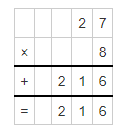Question 2.
7,365 × 9 = ___
7,365 × 9 = 66,285.

Explanation:
The multiplication of 7,365 × 9 is 66,285.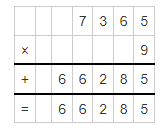Question 3.
94 × 67 = ___
94 × 67 = 6298.

Explanation:
The multiplication of 94 × 67 is 6,298.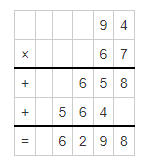Question 4.
827 × 61 = ___
827 × 61 = 50,447.

Explanation:
The multiplication of 827 × 61 is 50,447.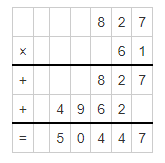Question 5.
625 × 29 = ___
625 × 29 = 18,125.

Explanation:
The multiplication of 625 × 29 is 18,125.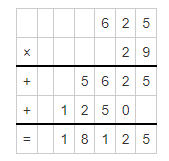Question 6.
944 × 38 = ___
944 × 38 = 35,872.

Explanation:
The multiplication of 944 × 38 is 35,872.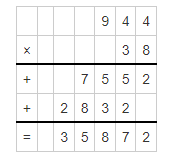Divide. (Lessons 3.3 and 3.4)

Question 7.
216 ÷ 3 = __
216 ÷ 3 = 72.

Explanation:
The division of 216 ÷ 3 is 72.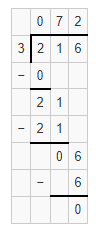Question 8.
432 ÷ 8 = ___
432 ÷ 8 = 54.

Explanation:
The division of 432 ÷ 8 is 54.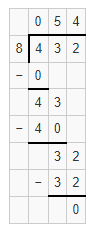Question 9.
5,520 ÷ 6 = __
5,520 ÷ 6 = 920.

Explanation:
The division of 5,520 ÷ 6 is 920.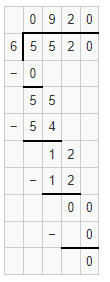Question 10.
2,828 ÷ 7 = ___
2,828 ÷ 7 = 404.

Explanation:
The division of 2,828 ÷ 7 is 404.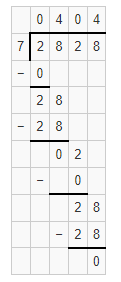Question 11.
5,398 ÷ 5 = ___
5,398 ÷ 5 = 1,079 R3.

Explanation:
The division of 5,398 ÷ 5 is 1,079 R3.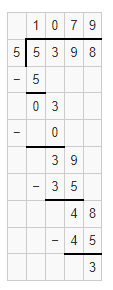Question 12.
7,436 ÷ 7 = ___
7,436 ÷ 7 = 1,062 R2.

Explanation:
The division of 7,436 ÷ 7 is 1,062 with Remainder as 2.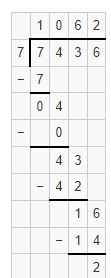Study the bar graph and answer the questions. (Lesson 4.1)

The bar graph shows the number of times Will wrote the letters A, B, C, D, and E on a paper.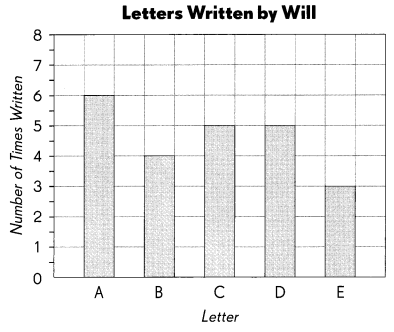complete the table. Use the data in the graph.

Question 13.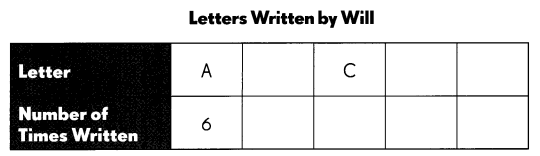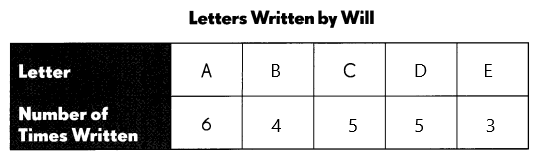Complete. Use the data in the table.

Question 14.
Which letter did Will write the greatest number of times? ____
A, 6 times.

Explanation:
The letter did Will wrote the greatest number of times is A which is 6 times.

Question 15.
How many more letter ‘A’s did Will write than the letter he wrote the least number of times? ___
3 times.

Explanation:
The number of more letters ‘A’s did Will write than the letter he wrote the least number of times is 3 more times s the least number is 3.

Question 16.
How many more letter ‘A’s must be written so that the number of letter ‘A’s will be 3 times the number of letter ‘B’s? ____

Explanation:
The number of more letter ‘A’s must be written so that the number of letter ‘A’s will be 3 times the number of letter ‘B’s is

Count the buttons and complete the table. (Lesson 4.1)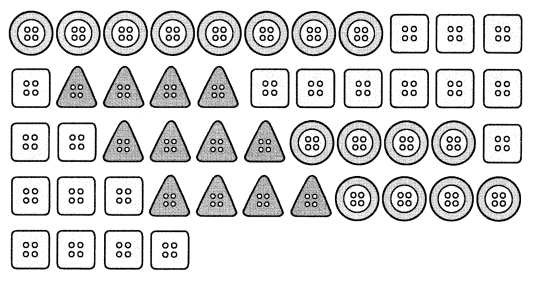Question 17.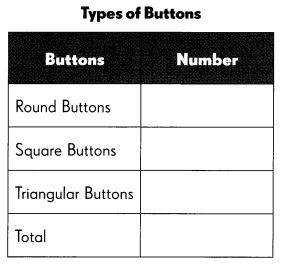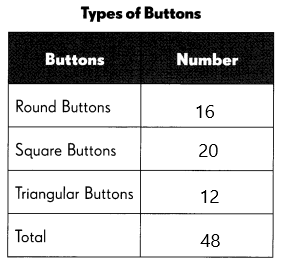Complete. Use the data in the table.

Question 18.
The least number of buttons are the ___ buttons.
Triangular buttons.

Explanation:
The least number of buttons are the triangular buttons which are 12.

Question 19.
There are ___ more square buttons than round buttons.
There are 4 more square buttons than round buttons.

Explanation:
The number of square buttons are 20 and the round buttons are 16. So the more square buttons than round buttons is 20-16 = 4 buttons.

Complete the table by finding the rows, columns, and intersections. (Lesson 4.2)

The table shows the types of sandwiches ordered by a group of students at lunchtime.

Question 20.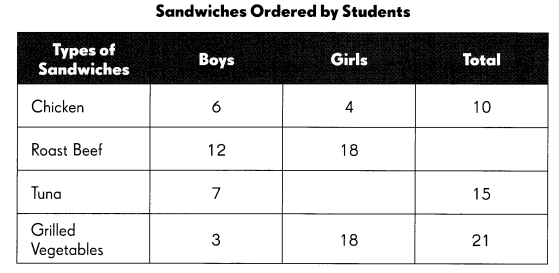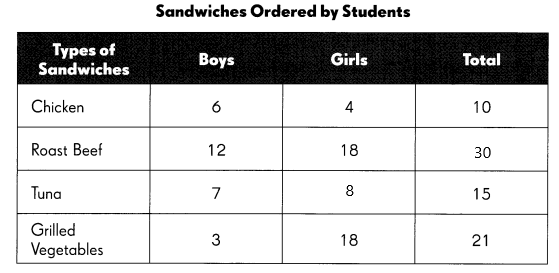Complete. Use the data in the table.

Question 21.
How many students ordered roost beef?
30 students.

Explanation:
The number of students who ordered roost beef is 30 students.

Question 22.
Find the number that should appear in the intersection for tuna’ and Girls’.

Question 23.
In which column does the number ‘7’ appear? ____
Boys column.

Explanation:
In the boy’s column, the number 7 appeared.

Question 24.
In which row does the number ‘6‘ appear? ____
Chicken sandwich.

Explanation:
In the chicken sandwich the number 6 appeared.

Question 25.
The number ‘4‘ appears in the intersection of the column for ____ and the row for ___.
Column for girls and the row for a chicken sandwich.

Explanation:
The number ‘4‘ appears at the intersection of the column for girls and the row for a chicken sandwich.

Complete the table by finding the rows, columns, and intersections. (Lesson 4.2)

The table shows the 50-cent and 20-cent toys that three friends bought for party favors.

Question 26.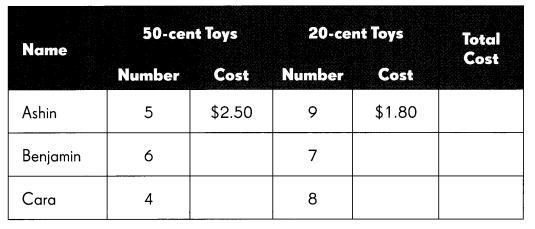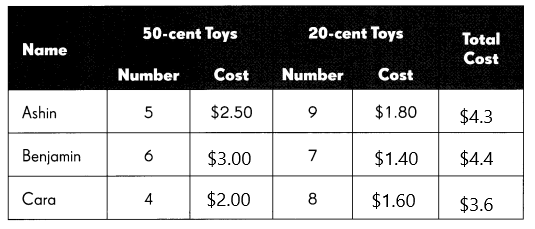Complete. Use the data in the table.

Question 27.
Who bought the most toys? ____
Cara.

Explanation:
Most of the toys are bought by Cara the total number is 12.

Question 28.
Who spent the most on the toys? ____
Benjamin.

Explanation:
Benjamin spent the most on the toys.

Question 29.
How much more did Benjamin spend than Cara? ___
Benjamin spends $0.8 than Cara Explanation: Benjamin spent on toys$4.4 and Cara spent $3.6, so Benjamin spends$4.4-$3.6 which is$0.8.

Question 30.
How much did they spend on 20-cent toys altogether? ____
The total money spends on 20-cent toys altogether is $4.80. Explanation: Total 20-cent toys are 24, so the total money spend on 20-cent toys altogether is 24×20 cents which is$4.80.

Question 31.
How much more did they spend on 50-cent toys than on 20-cent toys? ___
The total money spends on 50-cent toys altogether is $7.50. Explanation: Total 50-cent toys are 15, so the total money spend on 50-cent toys altogether is 15×50 cents which is$7.50.

Complete. Use the data in the line graph. (Lesson 4.3)

The graph shows the amount of water in a leaking tank over 7 hours.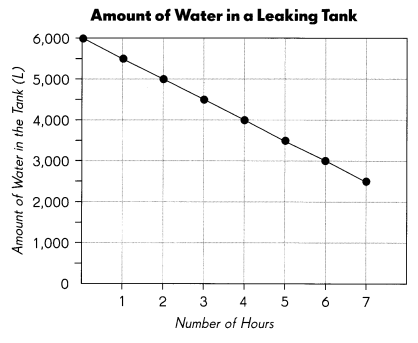Question 32.
What was the amount of water in the tank at the start? _______
6,000 liters.

Explanation:
The amount of water in the tank at the start is 6,000 liters.

Question 33.
What was the amount of water in the tank after 7 hours? _____
2,500 liters.

Explanation:
The amount of water in the tank after 7 hours is 2,500 liters.

Question 34.
After how many hours was the amount of water in the tank half that at the start? ___________
6 hours.

Explanation:
It took 6 hours to the amount of water in the tank half that at the start.

Question 35.
The owner of the tank paid a fine of $1 for every 8 liters of water lost. How much would the fine be after 4 hours? ________ Answer: The owner needs to pay$500.

Explanation:
After 4 hours the amount of water lost is 4,000 liters. As the owner needs to pay a fine of $1 for every 8 liters of water lost. So the owner needs to pay 4,000÷8 which is$500.

Complete. Use the data in the line graph. (Lesson 4.3)

The line graph shows the change in water level in a tank over 6 minutes.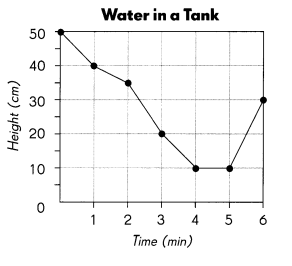Question 36.
What was the height of the water after
a. 2 minutes? ____
35 cm.

Explanation:
The height of the water after 2 minutes is 35 cm.

b. 3$$\frac{1}{2}$$ minutes? ____
15 cm.

Explanation:
The height of the water after 3$$\frac{1}{2}$$ minutes is 15 cm.

Question 37.
What was the decrease in the height of the water from the first to the second minute? ___
The decrease in the height of the water from the first to the second minute is 5 cm.

Explanation:
The height of the water in the first minute is 40 cm and the height of the water in the second minute is 35 cm. So the decreased height is 40-35 which is 5 cm.

Question 38.
During which 1 -minute interval did the water level decrease the most?
From the ___ minute to the ___ minute.
From the 2nd minute to the 3rd minute.

Explanation:
From the 2nd minute to the 3rd minute the water level decreases the most.

Question 39.
During which 1 -minute interval did the water level increase by 20 centimeters?
From the ___ minute to the ___ minute.
From the 5th minute to the 6th minute.

Explanation:
From the 5th minute to the 6th minute the water level increased by 20 centimeters.

Question 40.
Was the tank ever empty? ____
If the tank were ever empty, how would you tell from the graph?

Problem Solving

Question 41.
Mr. Suarez has $2,760 to buy family meals for the local food pantry. a. What is the greatest number of family meals he can buy if each meal costs$9?
The greatest number of family meals Mr. Suarez bought is 306 meals.

Explanation:
The greatest number of family meals he can bought if each meal costs $9 is$2,760÷$9 which is approx 306 meals. b. How much money would he have left after buying the meals? Answer: The money left with Mr. Suarez is$6.

Explanation:
The money did Mr. Suarez had is $2,760, if he bought 306 meals it will be 306×$9 which is $2,754. So the money left with Mr. Suarez will be$2,760-$2,754 which is$6.

Question 42.
A grocer bought two bags of dried fruit. One bag contained 4,950 ounces of fruit and the other bag contained 2,730 ounces of fruit. He repacked the fruit equally into 8 smaller packets. What was the weight of the fruit in each packet?
The weight of the fruit in each packet is 960 ounces.

Explanation:
Given that a grocer bought two bags of dried fruits and one bag contained 4,950 ounces of fruit and the other bag contained 2,730 ounces of fruit, so the total will be 4,950+2,730 = 7,680 ounces. He repacked the fruit equally into 8 smaller packets. So the weight of the fruit in each packet is 7,680÷8 which is 960 ounces.

Question 43.
A farmer packed 37 pumpkins. Each pumpkin had a weight of about 48 ounces. He put them into three baskets.

• The weight of the pumpkins in Basket A was 3 times that of the pumpkins in Basket C.
• The weight of the pumpkins in Basket B was twice that of the pumpkins in Basket C.
• The weight of the empty Basket C was 140 ounces.

What was the total weight of Basket C and the pumpkins in it?
The total weight of Basket C and the pumpkins in it is 436 ounces.

Explanation:
Given that a farmer packed 37 pumpkins and each pumpkin had a weight of about 48 ounces. So the total weight of the pumpkins is 37×48 which is 1,776 ounces.
Let the weight of Basket C be X, as Basket A was 3 times that of the pumpkins in Basket C
and the weight of Basket A be 3X, the eight of the pumpkins in Basket B was twice that of the pumpkins in Basket C,
the weight of Basket B will be 2X. So the total weight of pumpkins is
3X+2X+X = 1,776 ounces,
6X = 1,776 ounces,
X = 1,776 ÷ 6
= 296 ounces.
As the weight of the empty Basket C was 140 ounces.
So the total weight of Basket C and the pumpkins in it is 296+140 which is 436 ounces.

Question 44.
The tank at a gas station contained 400 gallons of gas. A tanker truck that contained 8,100 gallons of gas filled the station’s tank. After that the tanker truck had 4 times as much gas as the station’s tank. How much gas did the tanker truck put into the station’s tank?